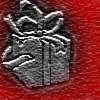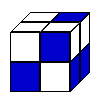#### You may also like### Cubes

How many faces can you see when you arrange these three cubes in different ways?### Wrapping Presents

Choose a box and work out the smallest rectangle of paper needed to wrap it so that it is completely covered.### Cubic Conundrum

Which of the following cubes can be made from these nets?

# The Big Cheese

##### Age 7 to 11Challenge Level

Chloe, Emily, Rebecca, Scarlett, Chloe, Alana, Isobel, Shauna and Joel from Griffithstown Primary School sent in various solutions that were all very interesting and showed hard work. Here is a summary taken from their suggestions.

I found out that we can share the $13$ slices of the big cheese between $5$ people. This is how we did it:
The first person had a slice of cheese that was $25$cm$^2$.
The second person had $3$ slices of cheese that was $20$cm$^2$ + $4$cm$^2$ + $1$cm$^2$ = $25$cm$^2$.
The third person had $5$ slices of cheese that was $12$cm$^2$ + $6$cm$^2$ + $4$cm$^2$ + $2$cm$^2$ + $1$cm$^2$= $25$cm$^2$.
The fourth person had $2$ slices of cheese that was $16$cm$^2$ + $9$cm$^2$ = $25$cm$^2$.
The fifth person had $2$ slices of cheese that was $16$cm$^2$ + $9$cm$^2$ = $25$cm$^2$.
So everybody had an equal share of cheese but in different sizes.

Cherian from Quarry Bay School sent in the following;

Solution for the third investigation for The Big Cheese. My solution:
First you imagine the two $1$ by $1$ by $1$ cubes put on top of each other.
Then you put the $1$ by $1$ by $2$ layer beside the other one.
After that, put another $1$ by $1$ by $2$ layer in front of it, so that you will have a $2$ by $2$ by $2$ cube.
Now you have to put $2$ by $2$ by $1$ layer on top of it.
Then you put $2$ by $3$ by $1$ layer on the left side.
After that you put a $3$ by $3$ by $1$ layer behind it.
Then you put another one on your cube.
Now you put a $3$ by $4$ by $1$ layer beside it.
Then put a $4$ by $4$ by $1$ layer behind it.
Now you have a $4$ by $4$ by $4$ cube.
After that you put a $4$ by $4$ by $4$ layer on top of it.
Then put a $4$ by $5$ by $1$ layer beside it.
Now put a $5$ by $5$ by $5$ layer behind it, so now you have a $5$ by $5$ by $5$ cube!!